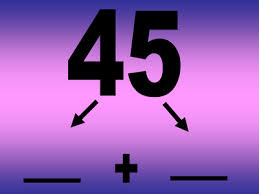# Game Of Partitions

Logic Level 2Alice and Carla are playing a game of their own invention. To both players, a positive integer $N > 1$ is known. Then, the game proceeds as follows:

1. Carla makes the first move by splitting $N$ into two (not necessarily distinct) positive integers that add up to it.

2. The next person picks one of the numbers (greater than 1) and further splits that number into two more positive integers.

3. If one of the numbers split is a 1, the player gets a point. (If both are 1, the player gets two points.)

4. The next person takes their turn by repeating steps 2 and 3 until there are only $N$ ones, meaning there are no longer any numbers that can be split.

If both Alice and Carla play optimally, given a positive integer $N$ it can be determined who should win the game. For how many positive integers $1 < N \leq 100$ should Carla be the winner?

Example Game:

Carla chooses the number 5 - Numbers: {5}

Carla splits 5 into 2 and 3 - Numbers: {2,3}

Alice splits the 2 into 1 and 1 and scores two points - Numbers: {1,1,3}

Carla splits the 3 into 1 and 2 and scores one point - Numbers: {1,1,1,2}

Alice splits the 2 into 1 and 1 and scores two points - Numbers: {1,1,1,1,1}

The numbers have been split into 5 ones, therefore the game ends 1-4 in favor of Alice.

×

Problem Loading...

Note Loading...

Set Loading...